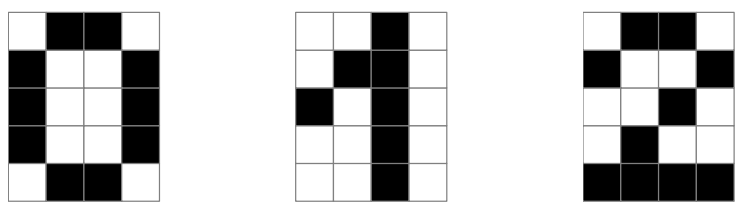시간 제한 메모리 제한 제출 정답 맞은 사람 정답 비율
1 초 128 MB 0 0 0 0.000%

## 문제

Irene works for Novel Efforts in Effective Recognition of Characters (NEERC). Her new project concerns image recognition using robots.

Since the approach is quite innovative, Irene starts with a very simple model first. She fixed $d$ images which are called digits $0$ to $d - 1$. Each image is a $w \times h$ rectangle filled with white and black unit squares (call them pixels). All images are distinct (that is, each two images differ in at least one pixel).

The robot is placed in the upper left pixel of one of the images. It starts executing a program written in a specific programming language described below. The task of the robot is to recognize which of the $d$ images it was placed onto.

The programming language for the robot consists of the following commands:

• 'U', 'D', 'L', 'R' --- movement commands. The robot moves one pixel up, down, left, or right respectively. If a movement command moves robot outside the image, the task is failed.
• '(' $\langle subprogram_w \rangle$ ':' $\langle subprogram_b \rangle$ ')' --- conditional operator. The robot checks the color of the pixel underneath itself. If it is white then $\langle subprogram_w \rangle$ is executed, otherwise $\langle subprogram_b \rangle$ is executed.
• '0', '1', ..., '9' --- recognized image commands. The robot must execute one of these commands when it knows which image it was placed onto. After such command, the program terminates.

Each movement command takes one time unit to execute. The execution of conditional operator and image recognized commands is instantaneous.

Irene is interested in the program that always works correctly. That is, if a robot is placed onto the image corresponding to the digit $i$, then the execution of the program must end with the command 'i'.

Given the set of images, design a correct program for the robot, such that its execution time in the worst case is minimal.

## 입력

The first line contains three integers $d$, $h$, and $w$ ($1 \le d \le 10$; $1 \le h, w \le 10$) --- the number of considered images, the height and the width of each image.

The rest if the input file contains $d$ descriptions of images. Each description consists of $h$ lines of length $w$. All characters are either B or W, representing a black or a white pixel respectively.

Image descriptions are given in the order from $0$ to $d - 1$. Descriptions are separated by an empty line.

## 출력

Return a correct program for the robot with minimal possible worst-case execution time. If there are multiple possible programs, output any of them.

All whitespace is ignored when parsing a program.

## 예제 입력 1

3 5 4
WBBW
BWWB
BWWB
BWWB
WBBW

WWBW
WBBW
BWBW
WWBW
WWBW

WBBW
BWWB
WWBW
WBWW
BBBB


## 예제 출력 1

D(1:D(2:0))


## 힌트The robot has to distinguish between these three images in the example.